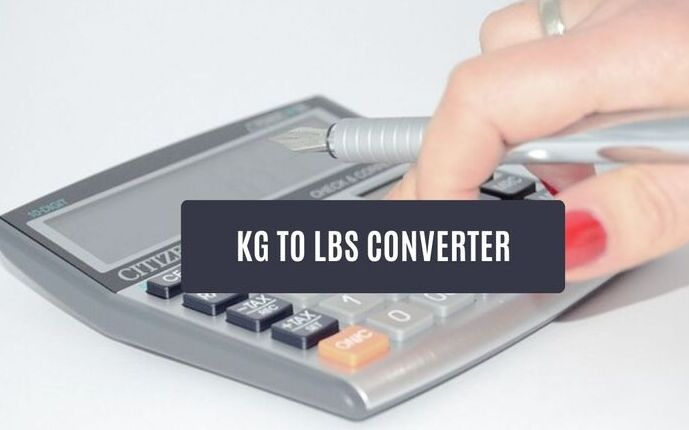December 7, 2023

# Kg to Lbs | Convert Kilograms to Pounds in Seconds## Kilograms To Pounds (kg to lbs ) Conversion Tool

Use our tool to convert Kilograms into Pounds in seconds. All you need to do is to provide a value in kilograms in the field provided and click on convert, and you will get the result in pounds(lbs).

Convertor

Kilogram is Lbs

## Formula to Convert Kg to Lbs

Since 1 kilogram is 2.20462 Pounds, you just need to multiply any number you want to convert into Pounds by 2.20462.

Formula:

Pounds = kilograms * 2.20462

For example, suppose you want to convert 5 kg to lbs. Then all you need to do is to multiply five by 2.20462

Pounds: 5 * 2.20462 = 11.0231

## Kilograms to Pounds conversion table

### Conclusion:

One kilogram (kg) is equal to 2.20462262185 pounds (lbs). To convert kilograms to pounds, just multiply the value of the kilogram by 2.20462, and you will get the desired result. You can also use our kg to lbs converter tools to convert easily in seconds.

## FAQs About Kilograms (kg) To Pounds (lbs) Conversion

1 Kilograms is equal to 2.20462 Pounds
5 kg is equal to 11.0231 Pounds
100 Kg is equivalent to 220.462 Pounds